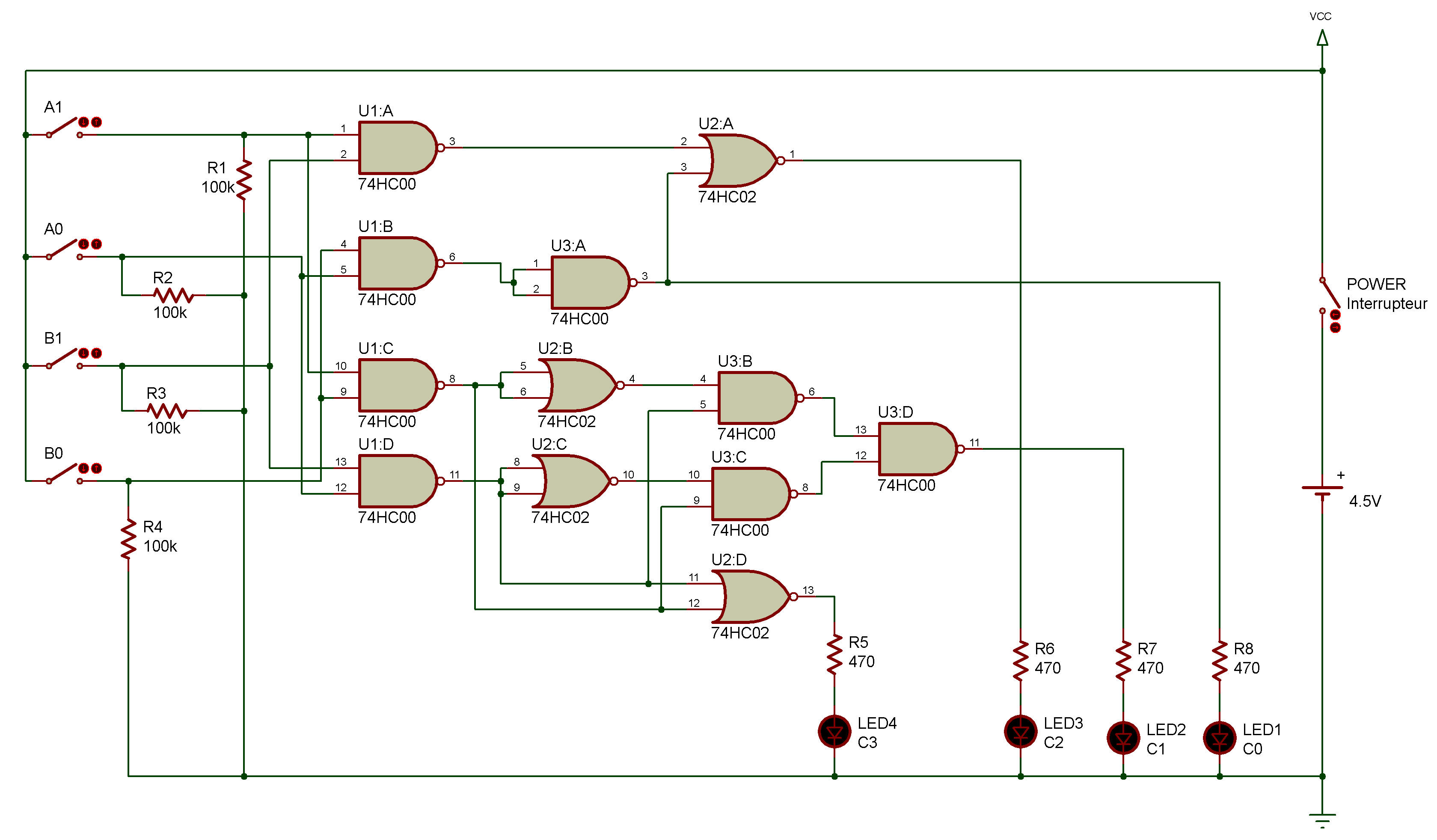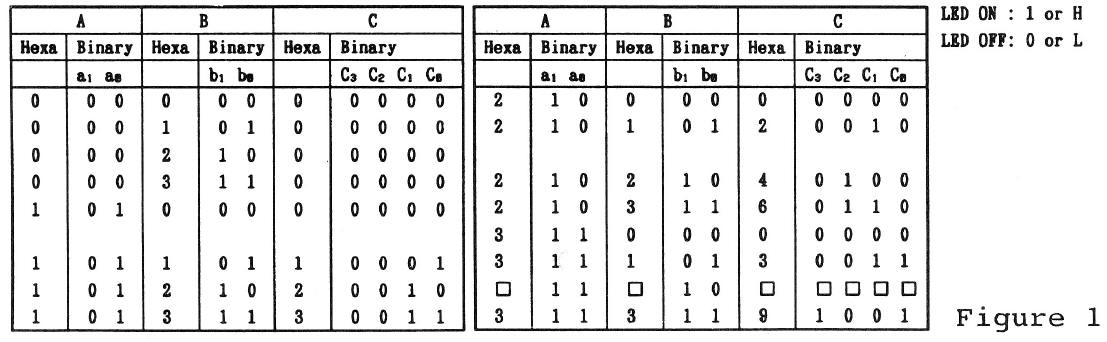Multiplier
Electronic

74HC00, 74HC02Here's a multiplier that can multiply of two numbers. You'll see from the schematic that the two numbers that can be multiplied by this circuit are input by A1 and A0 and the other by B1 and B0.

This means that each number is expressed by two bits, so the input numbers are limited to 0 - 3. Figure 1 shows the input number combinations and the results of multiplication. Output is expressed by 4-bit binary numbers produced by C0-C3 and displayed by LED 1 - LED 4.When you finish assembling, turn power ON and see how this multiplier works. Remember that 1 is indicated when any of A1, A0, B1 and B0 is ON and 0 when OFF.

An example of multiplication:

When you input 1 (a1 = 0, a0 = 1) to A and 3 (b1 = 1, b0 = 1) to B, only LED 1 and LED 2 light up.

Check this ON-OFF combination in Figure 1 and you'll find out that it means 3 which is the right answer. See how this multiplier circuit functions?

Now input a1 = 0, a0 = 1, b1 = 1 and b0 = 0, and write down the answer in the blank columns in Figure 1.

Recherche personnalisée